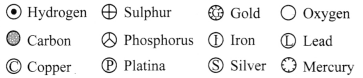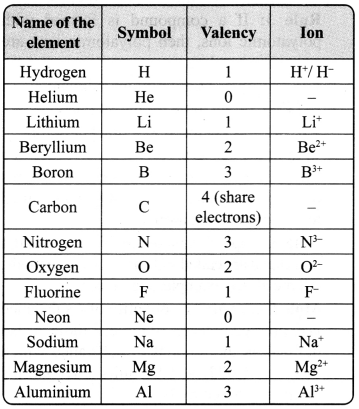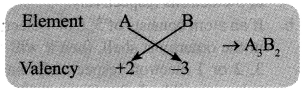## JAC Class 9 Science Notes Chapter 3 Atoms and Molecules

### JAC Board Class 9th Science Chapter 3 Notes Atoms and Molecules

→ In 430 B.C., Democritus postulated that matter is made up of very small particles calLed “Atomos’ which means ‘indivisible’. Later, Antoine Lavoisier, from his experimental observations, established the laws of chemical combinations.

→ The Law of Conservation of Mass: This law of conservation of mass states that, the total mass of reactants is equal to the total mass of products.
For example:
A + B → C + D
(Reactants) → (Products)
Total mass of reactants = Total mass of products

→ Law of Definite Proportions: According to the law of definite proportions, in a chemical substance, the elements are always present in definite proportion by mass. For example. in water, the ratio of the mass of hydrogen to the mass of oxygen is always 1: 8.

→ After a series of experiments, Dalton concluded that all matter must be composed of tiny particles, which cannot be further divided. He called them atoms.→ “The smallest particle of an element is called an atom.”
Atomic radius is measured in nanometer (nm).
1nm = 1 × 109m
1 m = 109nm
Atomic radii of hydrogen atom 1 × 10-10 m

→ Dalton’s Postulates:

• Matter is made up of atoms.
• Atoms are indivisible and indestructible.
• Atoms of a given element are identical in mass and in properties.
• Compounds are formed by a combination of two or more same or different kinds of atoms and a chemical reaction is a rearrangement of atoms.
• Atoms of different elements have different masses and chemical properties.
• Atoms combine in the ratio of small whole numbers to form compounds.
• The relative number and kind of atoms are constant in a given compound.

→ Elements and symbols: Dalton proposed a scheme of notation to represent elements.→ Symbols for some elements as proposed by Dalton Symbols of some common elements

 Name of the element Latin name Symbol Hydrogen – H Helium – He Carbon – C Copper Cuprum Cu Cobalt – Co Chloride – Cl Cadmium – Cd Boron – B Barium – Ba Bromine – Br Bismuth – Bi Sodium Natrium ‘ Na Potassium Kalium K Iron Ferrum Fe Gold Aurum Au Silver Argentum Ag Mercury Hydrargyrum Hg

→ Molecule: A molecule is a group of two or more atoms that are chemically bonded together and form the smallest part of an element or a compound. This can exist independently and shows all the properties of that substance.→ Atomicity: The number of atoms constituting a molecule is referred to as its atomicity. Molecules of an element constitute same type of atoms. They may be monoatomic, diatomic or polyatomic.

 Name of the element Atomicity Molecular formula Helium Monoatomic He Neon Monoatomic Ne Argon Monoatomic Ar Krypton Monoatomic Kr Xenon Monoatomic Xe Radon Monoatomic Rn Hydrogen Diatomic H2 Chlorine Diatomic Cl2 Nitrogen Diatomic N2 Phosphorus Polyatomic (Tetra) P4 Sulphur Polyatomic (Octa) S8

→ Ion: Ion is a charged particle and can be positively or negatively charged. A positively charged particle in a molecule is called cation, for example, Na+, Ca2+. An anion is a negatively charged particle in a molecule, for example, F, Cl.

→ Valency: The combining capacity of an element is known as valency. The combining capacity of the atoms to form molecules either with same or different elements is defined as valency. Valency is used to find out the number of atoms of an element that will combine with the atom of another element to form a chemical compound.

Every atom wants to become stable. To do so, it may lose, gain or share electrons.
a. If an atom consists of 1,2 or 3 electrons in its valance shell, then its valency is 1, 2 or 3 respectively, and it will lose 1, 2 or 3 electrons respectively.
b. If an atom consists of 5, 6 or 7 electrons in the outermost shell, then it will gain 3, 2 or 1 electron respectively, and its valency will be 3, 2 or 1 respectively.
c. If an atom has 4 electrons in the outermost shell, then it will share these electrons and hence its valency will be 4.
d. If an atom has 8 electrons in the outermost shell, then its valency is 0.→ Variable valency: Some elements show more than one valency, hence, termed as variable valency elements.
For example:
Iron shows
valency II – Fe2+ (Ferrous ion)
valency III – Fe3+ (Ferric ion)

Copper shows
valency I – Cu+ (Cuprous ion)
valency II – Cu2+ (Cupric ion)→ Chemical Formulae: The chemical formula of a compound is a symbolic representation of its composition.
Rule 1: Cross multiply the valency of elements to form a compound.Rule 2: If compound consists of both metal and non-metal, then metal is written first. For example: In calcium chloride (CaCl2) and zinc sulphide (ZnS), Calcium and Zinc are metals, so they are written first, whereas chloride and sulphide are non-metals.

Rule 3: If a compound is formed with polyatomic ions, then polyatomic ions are written in brackets.
For example: In aluminium sulphate [Al2(SO4)3], the polyatomic sulphate ion, SO42- is enclosed in a bracket before writing the subscript 3.
Here, the bracket with a subscript 3 indicates that three sulphate groups (SO42-) are joined to two aluminium atoms.

Note: Compounds made up of a metal and a non-metal are called salts or ionic compounds. All the above examples are of salts.

→ Mole Concept: The quantity of a substance is expressed in terms of mole. 1 mole is also defined as the amount of substance which contains 6.022 × 1023 units (Avogadro’s number denoted by NA).
For example 1 mole of oxygen atoms represents 6.022 × 1023 atoms of oxygen and 5 moles of oxygen atoms contain 5 × 6.022 × 1023 atoms of oxygen.

→ Molar Mass: The mass of 1 mole of a substance is called molar mass. Atomic mass or molecular mass in gram is equal to molar mass.
For example: Atomic mass of Fe is 56 u. Molar mass of Fe is 56 g.
Atomic mass of H2O is 18 u. Molar mass of H2O is 18 g.
Number of moles = $$\frac{\text { Given mass }}{\text { Molar mass }}$$

For example, number of moles in 122 g of iron will be
$$\frac{\text { Given mass }}{\text { Molar mass }}=\frac{112}{56}$$ = 2 × 6.022 × 1023 atoms.